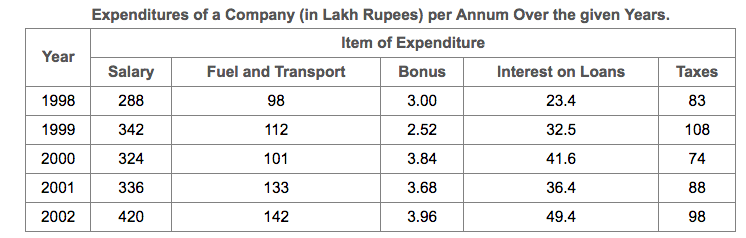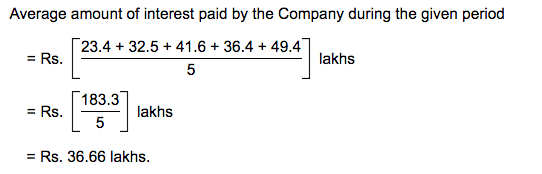# Tips and Tricks of Attempting DI questionsLast Updated on Nov 7, 2020

Data interpretation is the most scoring and time-consuming section in the competitive examinations. The DI section in competitive examinations can be rewarding but also your downfall if not attempted carefully. Here we have we have outlined some strategies that you can use to make sure that you attempt DI correctly.

### Strategies for Attempting DI

#### Read and Analyze the Question

• The first and the most important step in solving any Data Interpretation question is to read the question carefully
• The next step should be to analyze the given graph/data carefully
• Do not try to see the questions first and find out the answers accordingly. You will waste your time if you follow that method
• Try to understand the graph. What is it about? Which years does it cover? Is the data in absolute terms or in percentage terms? What do two-axis signify? Look at the statistics for each graph, chart, table or pie diagram. Look carefully at the labels. Make sure you understand the central theme of the data

### Take a call on attempting / not attempting:

• Some questions ask too much from you. They require a lot of calculation in order to be solved. These questions are known as the speed breakers. Such questions are best left alone, at least in the first round
• Once you have finished solving all the easy questions and still have time for the section to end, you should attempt these questions
• If you try such questions, you will lose your precious time on them and may not be able to attempt some simple questions that may follow

### Work on Speed Calculations

• DI is not about finding the exact answer always. Most of the times, the options given are far enough from each other to give you enough room for approximation
• So, for example, if you are asked to divide 542678/181234, you can easily approximate that the answer will be somewhere around 3 by looking at the first 2 numbers only. 54/18 is 3. So, instead of dividing it and finding the accurate answer, try to find an approximate answer. This will give you the correct answer more often than not

### Get acquainted with all the types of DI Questions

Familiarize yourself with all types of DI Questions. While practicing you will realize that there are different types/formats of DI Questions. For example pie charts, line graphs, bar charts, tables and so on. If you get familiar with all types of DI questions you will gain the confidence to solve all the types of questions in competitive exams.

### Patience and Persistence

There was a time when students use to hop from one set to another without completing one section. But now in the online test, you have no choice but to attempt all the questions in one section before jumping to other as you don’t have the option of returning back to the previous section.

#### Example-11.  What is the average amount of interest per year which the company had to pay during this period?
A. Rs. 32.43 lakhs
B. Rs. 33.72 lakhs
C. Rs. 34.18 lakhs
D. Rs. 36.66 lakhs

Solution### Summary

In this blog post. we have explained Strategies for Attempting DI, What to attempt and what not to attempt, how to increase your speed of calculations. The concept is clarified with the help of an easy example. For more posts on DI, click here.

Hope you liked this post. Share your comments in the comment section below.